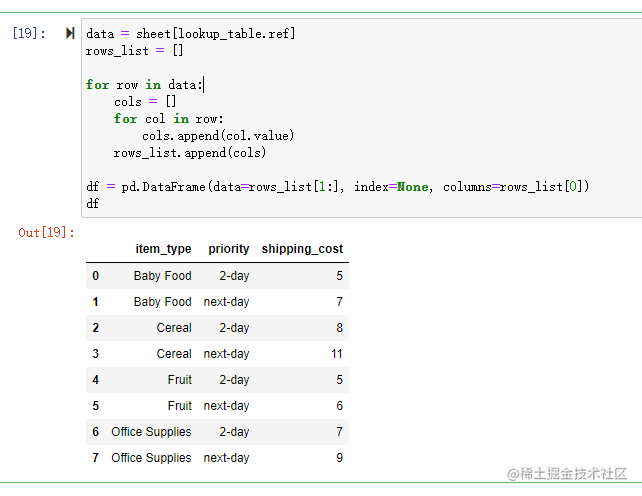## 两个使用 Pandas 读取结构不良 Excel 的方法，拿走不谢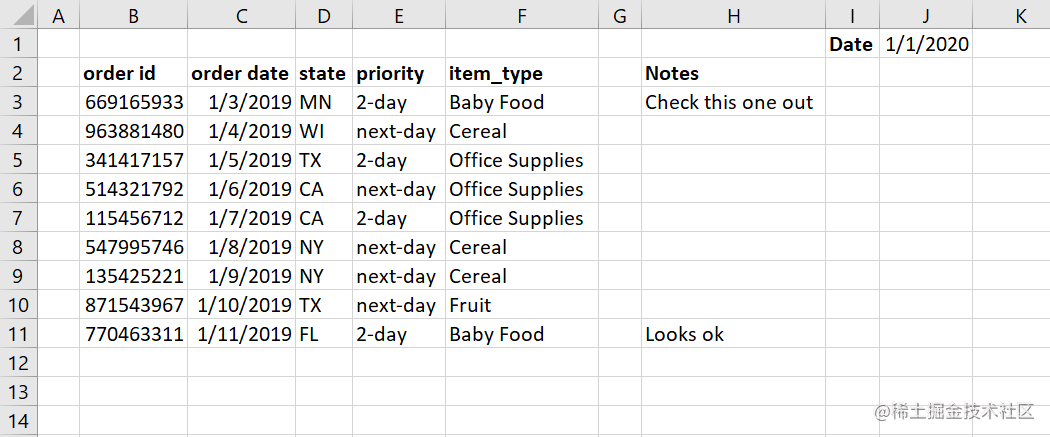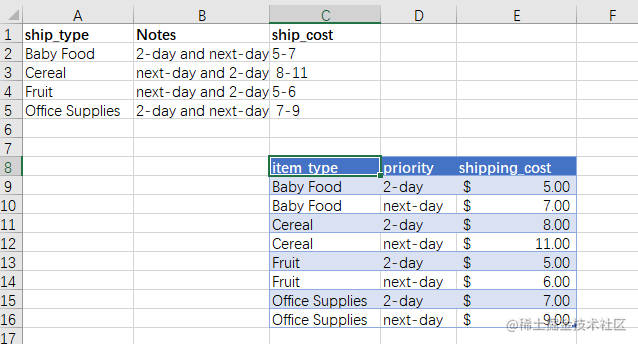# 指定列读取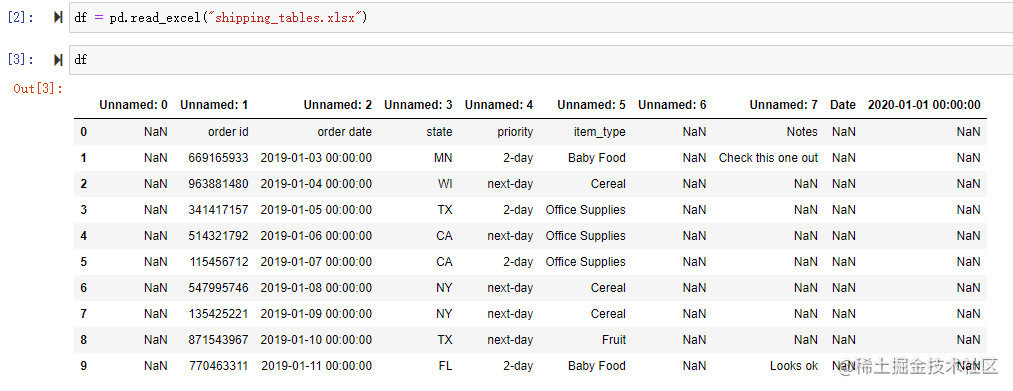``````from pathlib import Path
src_file = Path.cwd() /  'shipping_tables.xlsx'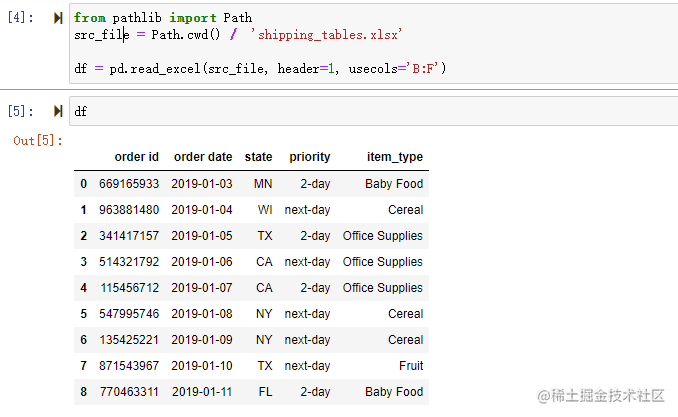可以看到生成的 DataFrame 中只包含我们需要的数据，特意排除了 notes 列和 date 字段

usecols 可以接受一个 Excel 列的范围，例如 B:F 并仅读取这些列，header 参数需要一个定义标题列的整数，它的索引从0开始，所以我们传入 1，也就是 Excel 中的第 2 行

``````df = pd.read_excel(src_file, header=1, usecols=[1,2,3,4,5])

``````df = pd.read_excel(
src_file,
usecols=['item_type', 'order id', 'order date', 'state', 'priority'])

``````def column_check(x):
if 'unnamed' in x.lower():
return False
if 'priority' in x.lower():
return False
if 'order' in x.lower():
return True
return True

``````cols_to_use = ['item_type', 'order id', 'order date', 'state', 'priority']
usecols=lambda x: x.lower() in cols_to_use)

# 范围和表格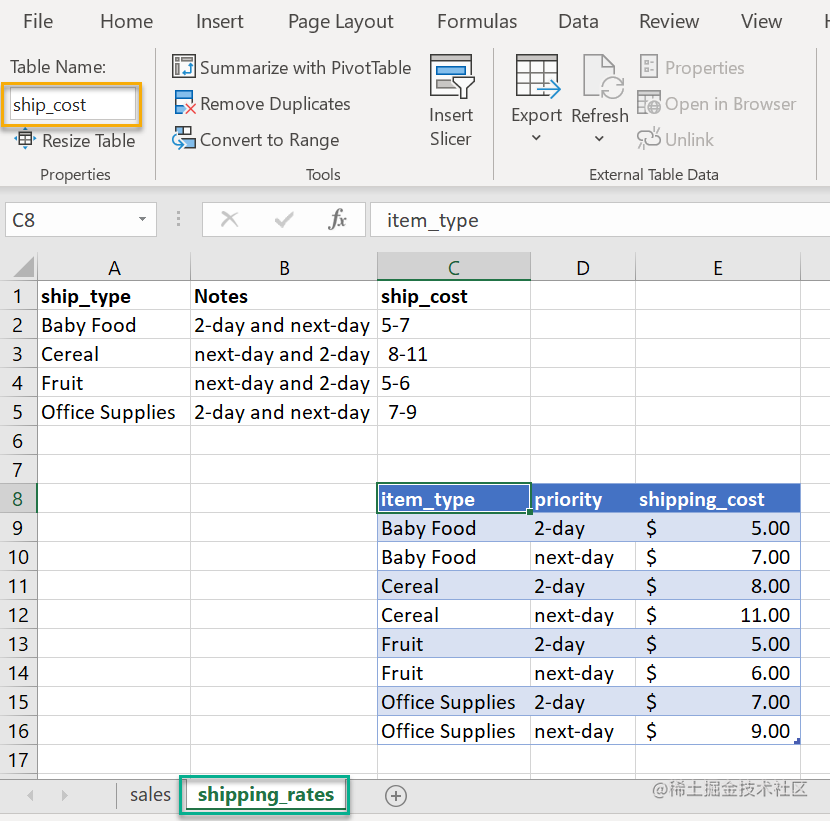``````from openpyxl import load_workbook
import pandas as pd
from pathlib import Path
src_file = src_file = Path.cwd() / 'shipping_tables.xlsx'

``````wb.sheetnames
sheet = wb['shipping_rates']
lookup_table = sheet.tables['ship_cost']
lookup_table.ref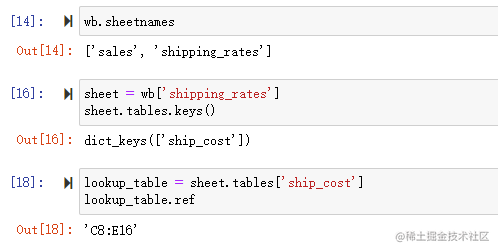``````# 获取数据范围
data = sheet[lookup_table.ref]
rows_list = []

# 循环获取数据
for row in data:
cols = []
for col in row:
cols.append(col.value)
rows_list.append(cols)

df = pd.DataFrame(data=rows_list[1:], index=None, columns=rows_list)